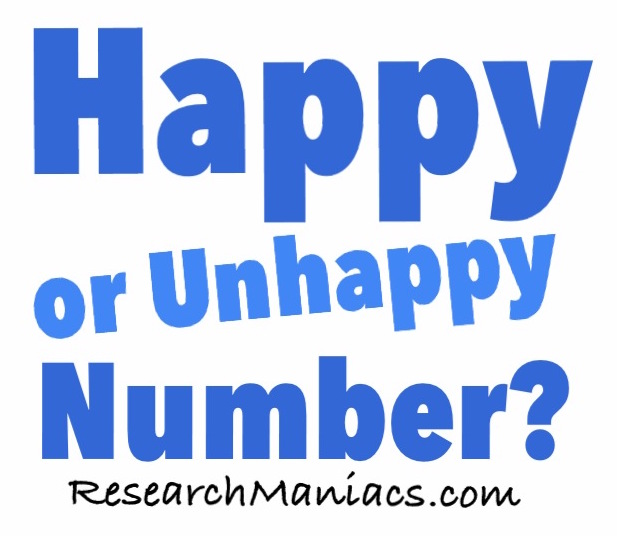Is 19 happy or unhappy number?

 To determine if 19 is a happy number or unhappy number, we square each digit in 19 and then add those numbers together. Then we repeat the process for the result until either the result turns to 1 or we realize it will never be 1. If it becomes a 1, then it is a happy numbers. Otherwise, it is an unhappy number. Here is the math to make it more clear: 12 + 92 = 8282 + 22 = 6862 + 82 = 10012 + 02 + 02 = 1 Since we ended up with a 1 at the end, 19 is a: Happy Number Is 20 happy or unhappy number? Do you think you can figure out if a number is happy or unhappy on your own know? Try the next number on our list and then check your answer here.Happy or Unhappy Number? Enter another number below to find out if it is happy or unhappy.